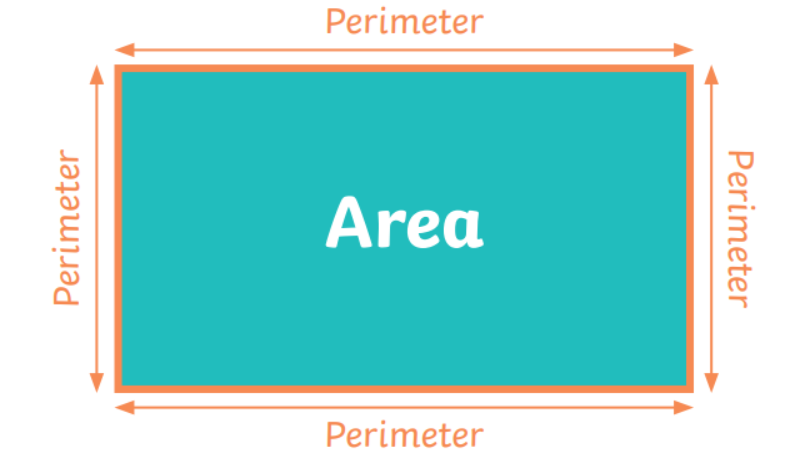# Maths - 7th February

Today we will be focusing on perimeter again.Year 3 - you will be focusing on calculating perimeter.

Here is the worksheet for today -

Y3-Spring-Block-4-WO8-Calculate-perimeter-2019
.pdf

Y3-Spring-Block-4-ANS8-Calculate-perimeter-2019
.pdf

Year 4 - you will be focusing on calculating the perimeter of a rectangle.

Here is the worksheet for today -

Y4-Autumn-Block-3-WO3-Perimeter-of-a-rectangle-2019
.pdf